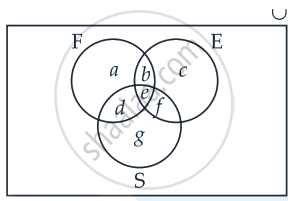# In a group of 50 students, the number of students studying French, English, Sanskrit were found to be as follows:French = 17, English = 13, Sanskrit = 15 French and English = 09, English and San - Mathematics

Diagram
Sum

In a group of 50 students, the number of students studying French, English, Sanskrit were found to be as follows:
French = 17, English = 13, Sanskrit = 15 French and English = 09, English and Sanskrit = 4 French and Sanskrit = 5, English, French and Sanskrit = 3. Find the number of students who study English only

#### Solution

Let us use Venn diagram method.Total number of students = 50

⇒ n(U) = 50

Number of students who study French = 17

⇒ n(F) = 17

Number of students who study English = 13

⇒ n(E) = 13

Number of students who study Sanskrit = 15

⇒ n(S) = 15

Number of students who study French and English = 9

⇒ n(F ∩ E) = 9

Number of students who study English and Sanskrit = 4

⇒ n(E ∩ S) = 4

Number of students who study French and Sanskrit = 5

⇒ n(F ∩ S) = 5

Number of students who study French, English and Sanskrit = 3

⇒ n(F ∩ E ∩ S) = 3

n(F) = 17

a + b + d + e = 17  ......(i)

n(E) = 13

b + c + e + f = 13   ......(ii)

n(S) = 15

d + e + f + g = 15   ......(iii)

n(F ∩ E) = 9

∴ b + e = 9   ......(iv)

n(E ∩ S) = 4

∴ e + f = 4   .......(v)

n(F ∩ S) = 5

∴ d + e = 5  ......(vi)

n(E ∩ F ∩ S) = 3

∴ e = 3   .......(vii)

From (iv)

b + 3 = 9

⇒ b = 9 – 3 = 6

From (v)

3 + f = 4

⇒ f = 4 – 3 = 1

From (vi)

d + 3 = 5

⇒ d = 5 – 3 = 2

Now from equation (i)

a + 6 + 2 + 3 = 17

⇒ a = 17 – 11

⇒ a = 6

Now from equation (ii)

6 + c + 3 + 1 = 13

⇒ c = 13 – 10

⇒ c = 3

From equation (iii)

2 + 3 + 1 + g = 15

⇒ g = 15 – 6

⇒ g = 9

Number of students who study English only, c = 3

Concept: Sets and Their Representations
Is there an error in this question or solution?

#### APPEARS IN

NCERT Mathematics Exemplar Class 11
Chapter 1 Sets
Exercise | Q 28.(ii) | Page 15

Share